# Knowledge Base

## Solution 11756: Finding the Sum, Difference, and Product of Two or More Matrices on the TI-89 Family, TI-92 Family, and Voyage™ 200 Graphing Calculators.

### How do I calculate the sum, difference, and product of matrices on the TI-89 family, TI-92 family, and Voyage 200 graphing calculators?

The examples below demonstrate how to calculate the sum, difference, and product of two or more matrices on the TI-89 family, TI-92 family, and Voyage 200.

Example: Find the sum, difference, and product of the following two matrices: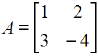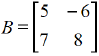Solution: First, enter matrices A and B:

1) On the TI-89 and TI-92 family, press [APPS]  .  On the TI-89 Titanium and Voyage 200, press [APPS] to display the Apps Desktop, then select Data/Matrix Editor App and press [ENTER], then press .
2) Set the type to Matrix, the name to "A" and the dimensions to 2 rows and 2 columns.
3) Press [ENTER] twice.
4) Input the above elements for matrix A into the Data/Matrix Editor screen, pressing [ENTER] after inputting each value.
5) Press [F1]  and set the type to Matrix, the name to "B" and the dimensions to 2 rows and 2 columns.
6) Press [ENTER] twice.
7) Input the above elements for matrix B into the Data/Matrix Editor screen, pressing [ENTER] after inputting each value.
8) After you have finished entering all the matrix elements, exit the Data/Matrix Editor App by pressing [HOME].

To add matrices A and B:

1) On the home screen, press [ALPHA] [A] [+] [ALPHA] [B]
2) Press [ENTER] to display the answer.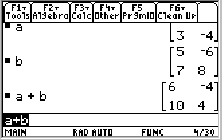To subtract matrices A and B:

1) On the home screen, press [ALPHA] [A] [-] [ALPHA] [B]
2) Press [ENTER] to display the answer.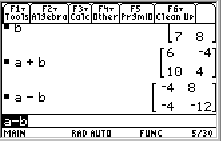To multiply matrices A and B:

1) On the home screen, press [ALPHA] [A] [x] [ALPHA] [B]
2) Press [ENTER] to display the answer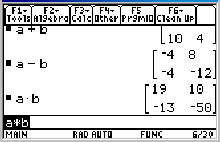For additional instructions on performing matrix operations, refer to the online guidebook for your calculator.